Build a recommendation engine with a restricted Boltzmann machine using TensorFlow

In this tutorial, learn how to build a restricted Boltzmann machine using TensorFlow that will give you recommendations based on movies that have been watched. The data sets used in the tutorial are from GroupLens, and contain movies, users, and movie ratings. You use a sigmoid activation function for the neural network, and the recommendations returned are based on the recommendation score that is generated by a restricted Boltzmann machine (RBM).

Learning objectives

In this tutorial, you’ll:

• Understand how restricted Boltzmann machines work
• Get to know how collaborative filtering can be implemented on restricted Boltzmann machines
• Understand the use cases and learning process of restricted Boltzmann machines

Prerequisites

The following prerequisites are required to follow the tutorial:

Estimated time

It should take you approximately 40 minutes to complete the tutorial.

What is a restricted Boltzmann machine?

A restricted Boltzmann machine is a two-layered (input layer and hidden layer) artificial neural network that learns a probability distribution based on a set of inputs. It is stochastic (non-deterministic), which helps solve different combination-based problems. RBMs can be used for dimensionality reduction, classification, regression, collaborative filtering, feature learning, and topic modeling.

As the name suggests, an RBM is a class of Boltzmann machines. However, they are restricted in certain ways when considering the connections between the input and the hidden nodes of the neural network, so it is easier to implement an RBM than a Boltzmann machine. The layers and the nodes within those layers are connected in a one-to-many fashion, where each node in the input layer is connected to every node in the hidden layer but no node within each layer is connected. The restriction allows more streamlined training algorithms than what is generally used in Boltzmann machines.

The following figure shows how an RBM looks. As you can see, all of the nodes in the input layer are connected to each node in the hidden layer. The structure of the neural network itself makes it efficient when training the neural network because one input layer can use many hidden layers for training. Multiple RBMs can be stacked on as well, creating a deep belief network that allows deeper learning of the neural network and incorporates further learning.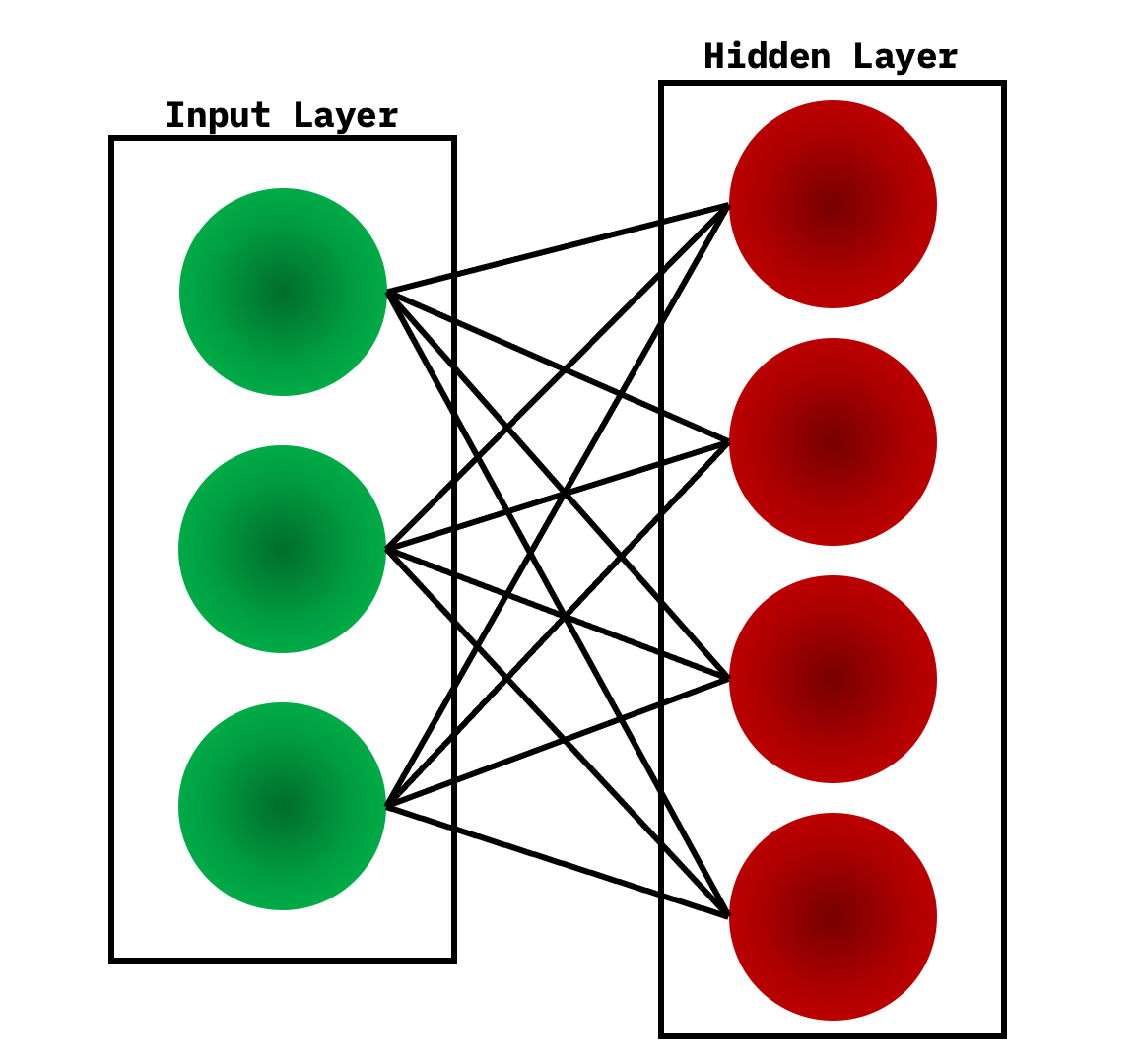How does a restricted Boltzmann machine work?

There are two steps involved when looking at how an RBM works: multiple inputs and reconstruction.

Multiple inputs

Multiple inputs are considered to be the first step when training the neural network. The inputs are taken into the input layer, multiplied by the weights, and added to the bias. After this, it goes through the activation function (sigmoid), and the outputs decide whether the hidden state gets activated.

The weights in the neural network are in a matrix, where the number of input nodes is the number of rows, and the number of hidden nodes is the number of columns. The primary hidden node obtains the vector multiplication of the inputs, and is multiplied by the first column of weights before the corresponding bias term is added to it.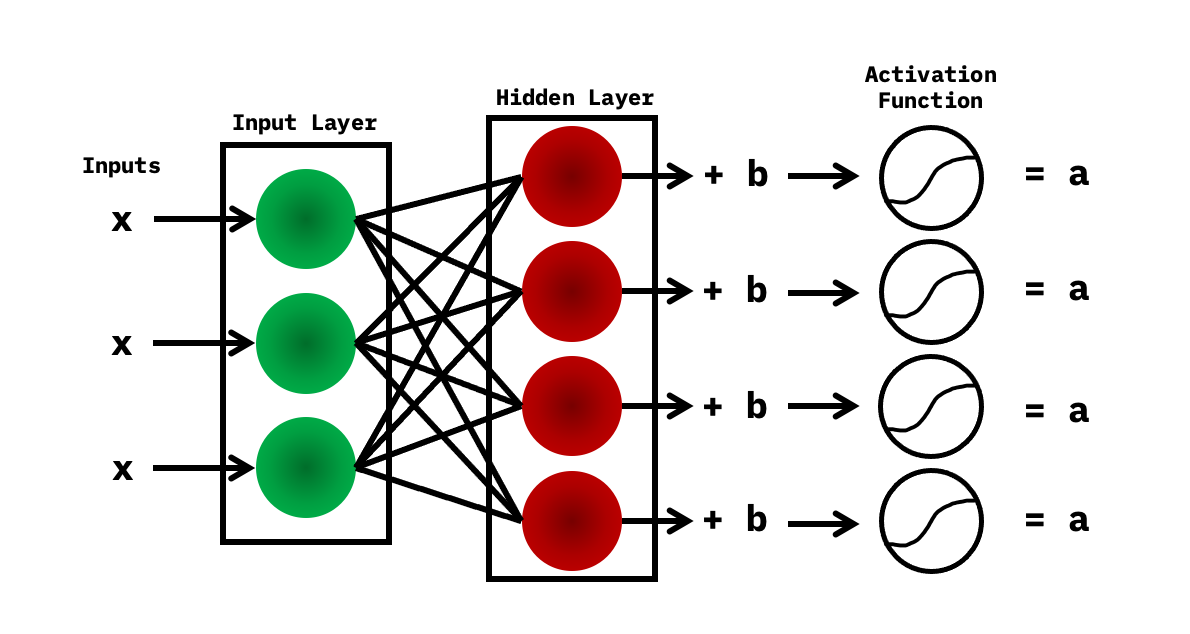Reconstruction

In reconstruction, the logic is pretty simple. You have the activations, which are the inputs at this point and are then passed to the hidden layer and then to the input later. After this, new biases are obtained, and the reconstruction is the new output.So how does the learning process really work? Because these two steps happen subsequently, you first generate activations using the multiple inputs phase, then reconstruction takes place. When the reconstruction is taking place in an epoch, the main goal is to decrease the reconstruction error so that the weights are then adjusted per iteration accordingly by the algorithm to decrease the reconstruction error. This gives you a good prediction and higher accuracy.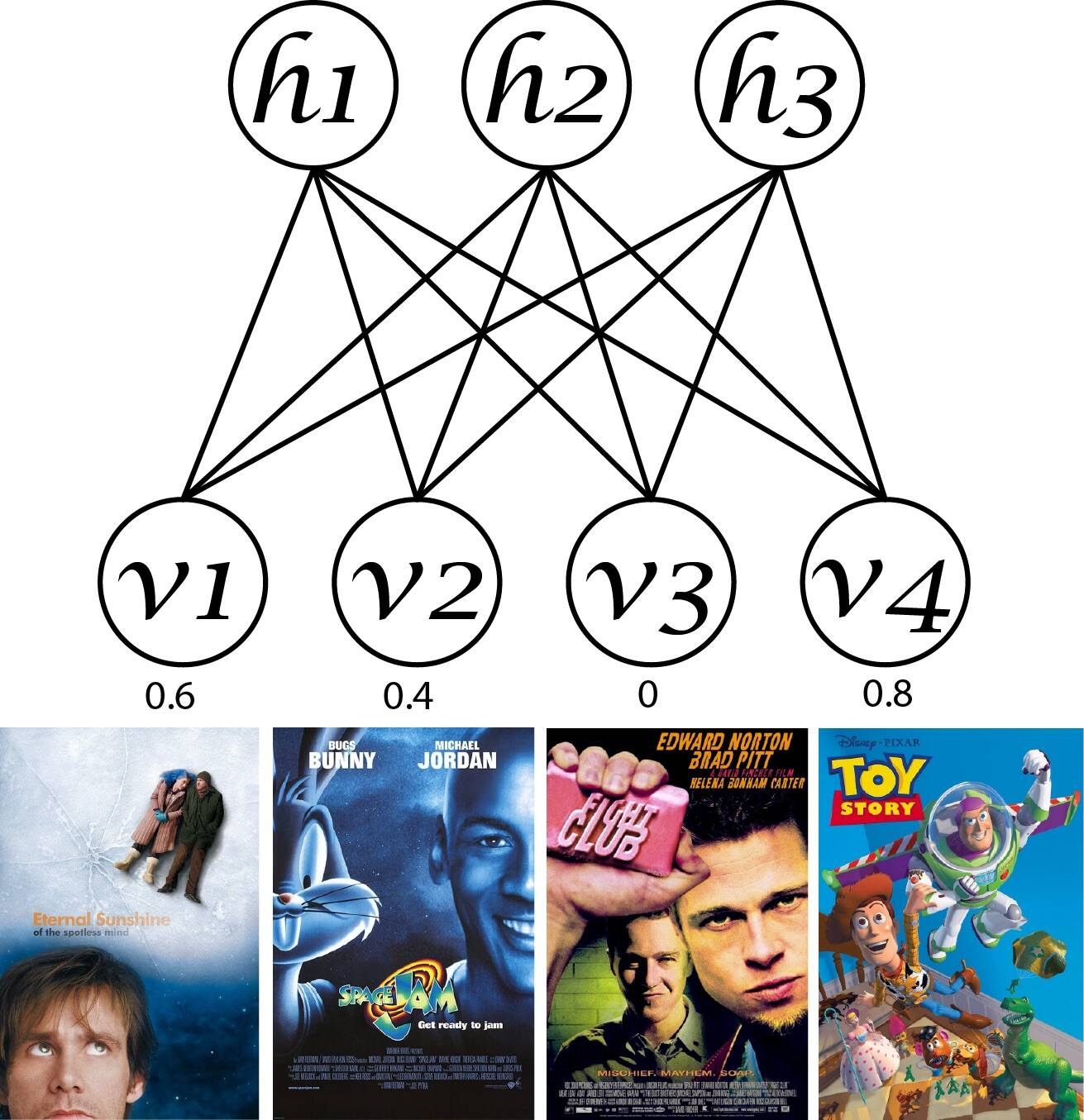Steps

Set up IBM Cloud Pak for Data as a Service

2. Create an instance of IBM Watson® Studio by searching for Watson Studio from the catalog.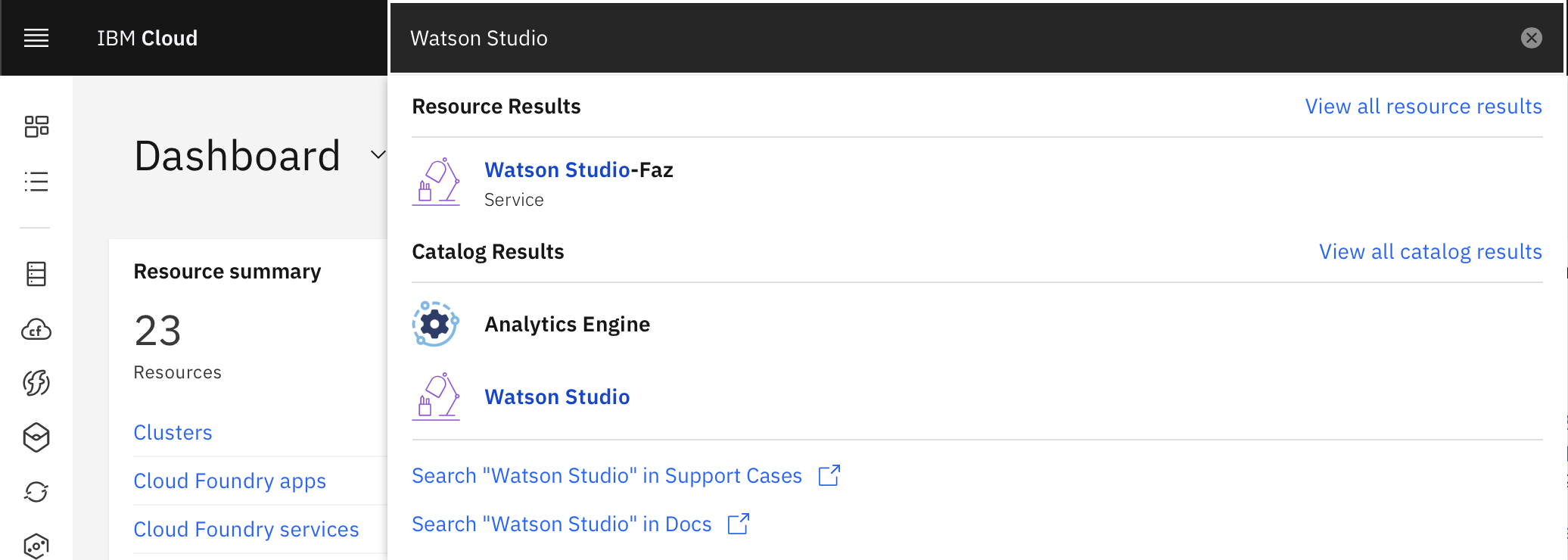3. Select the Lite plan, and click Create.Create a new project

1. Access the service, and click either Create a project or New project.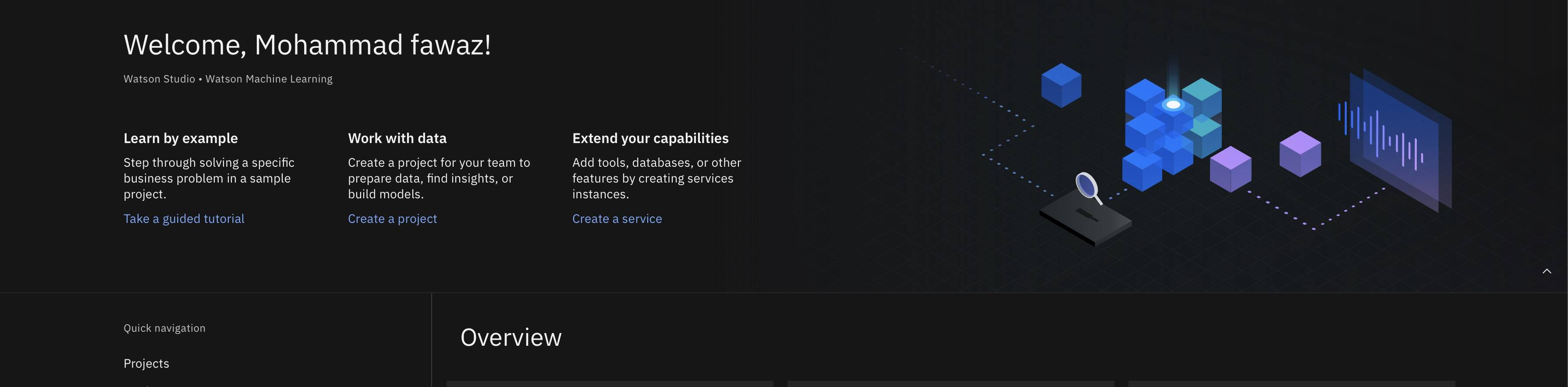2. Select Create an empty project.

3. Give the project a name.
4. Choose an existing IBM Cloud Object Storage service instance or create a new one.
5. Click Create.

Alternatively, you can click the navigation menu at the upper left, click View all projects, and create a new project.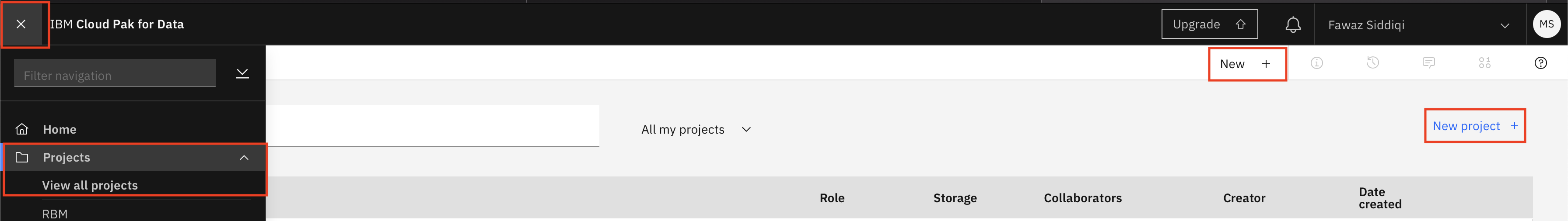Import the notebook

1. Access the created project.
2. Click Add to project +.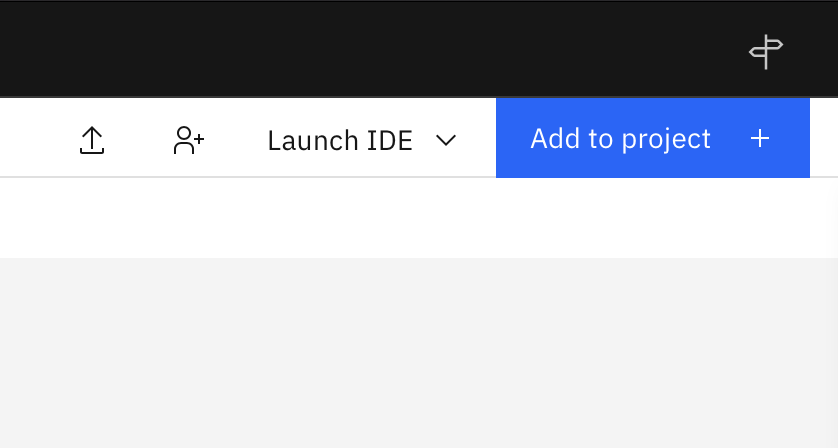3. Click Notebook.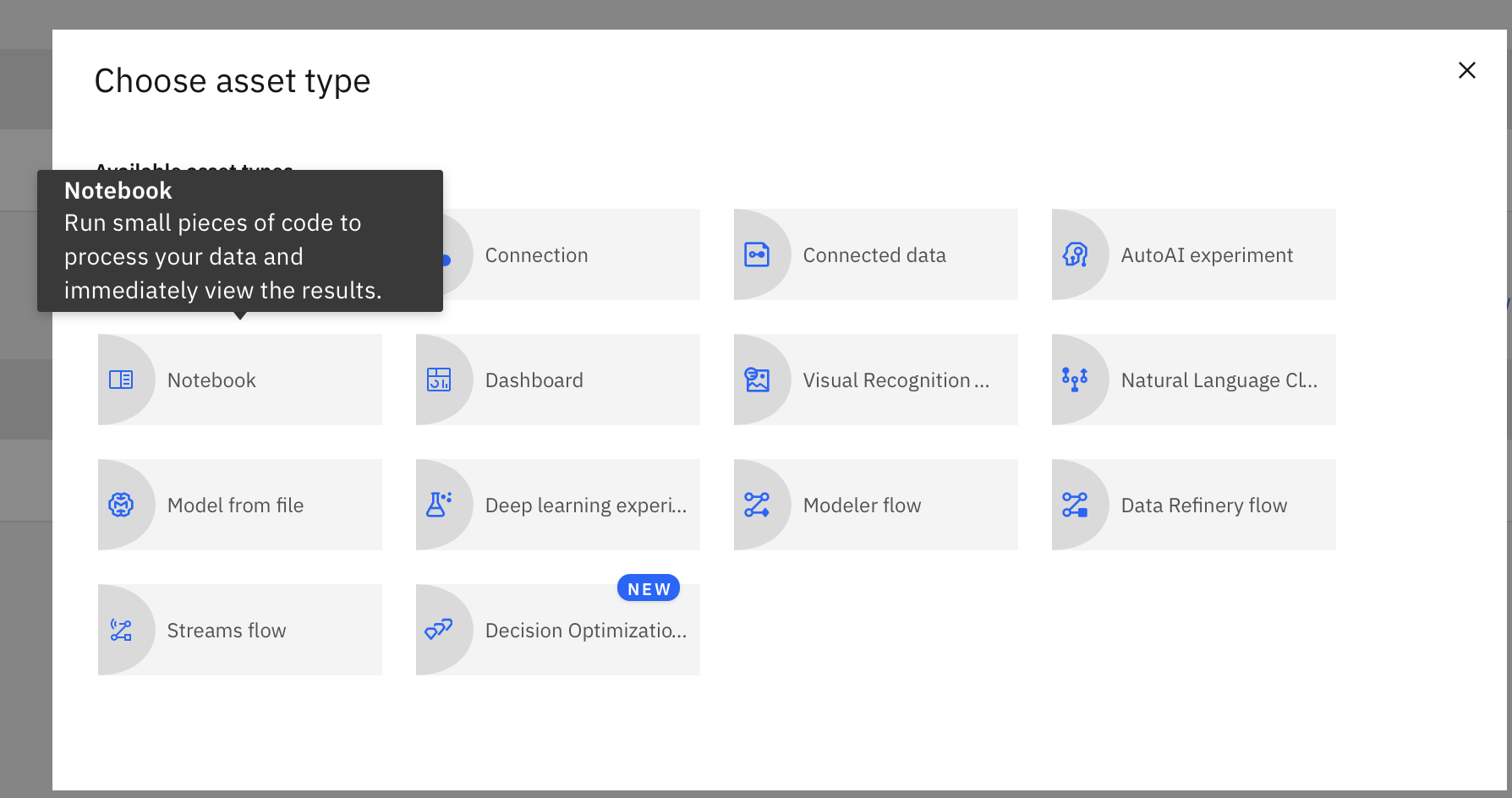4. Click From URL.

5. Name the notebook.
6. Under Select runtime, choose Default Python 3.7 XS.
7. Enter https://raw.githubusercontent.com/IBM/dl-learning-path-assets/main/unsupervised-deeplearning/notebooks/CollabortiveFilteringUsingRBM.ipynb as the Notebook URL.
8. Click Create.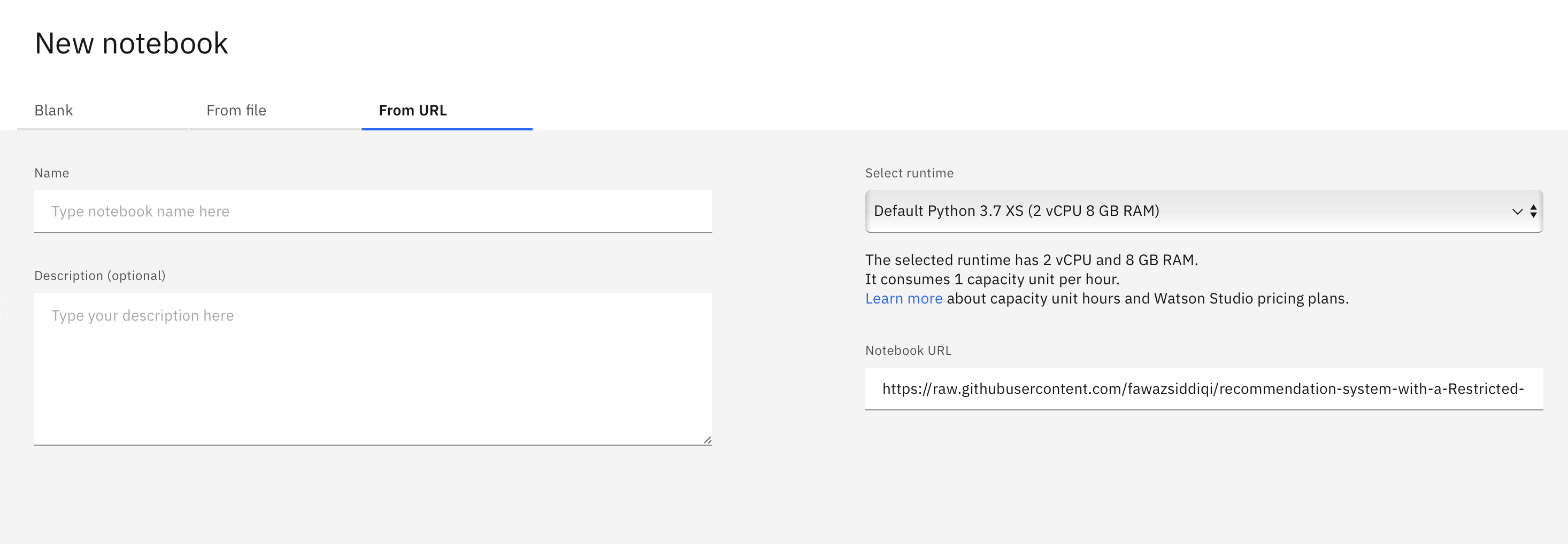9. Run the notebook. In the open notebook, click Run to run the cells one at a time. The rest of the tutorial follows the order of the notebook.Then, data is added into the data frame. In this example, you create two data frames movies_df for the movies and ratings_df for the movie ratings.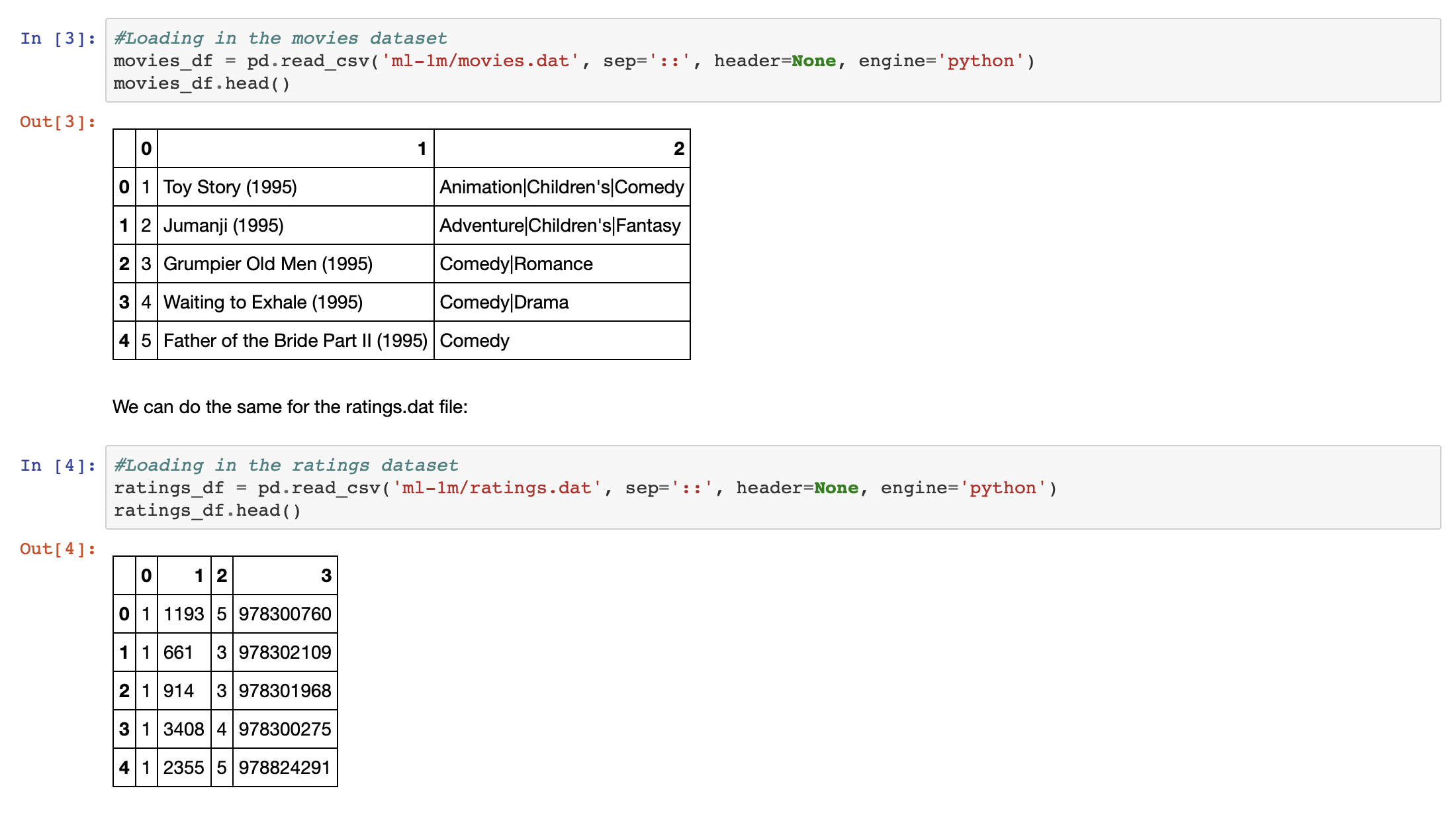You rename the columns in the data frames, making sure that you are able to understand the data properly.

• movies_df consists of three columns: MovieID, Title, and Genres
• ratings_df consists of four columns: UserID, MovieID, Rating, and TimestampTo normalize the data, when we create a pivot of ratings_df, there is a lot of data marked as NaN.

user_rating_df = ratings_df.pivot(index='UserID', columns='MovieID', values='Rating')

Therefore, we store the normalized users ratings as a matrix of user ratings called trX, and normalize the values.After normalizing the data, you set the model parameters, which includes the hidden units and the visible units. Then, you add the activation functions f.sigmoid and tf.relu because they are commonly used for RBMs, and continue with defining a function to return only the generated hidden states for the hidden layer and also for the reconstructed output.After the model parameters are set, you train the model with the following code:

epochs = 5
batchsize = 500
errors = []
weights = []
K=1
alpha = 0.1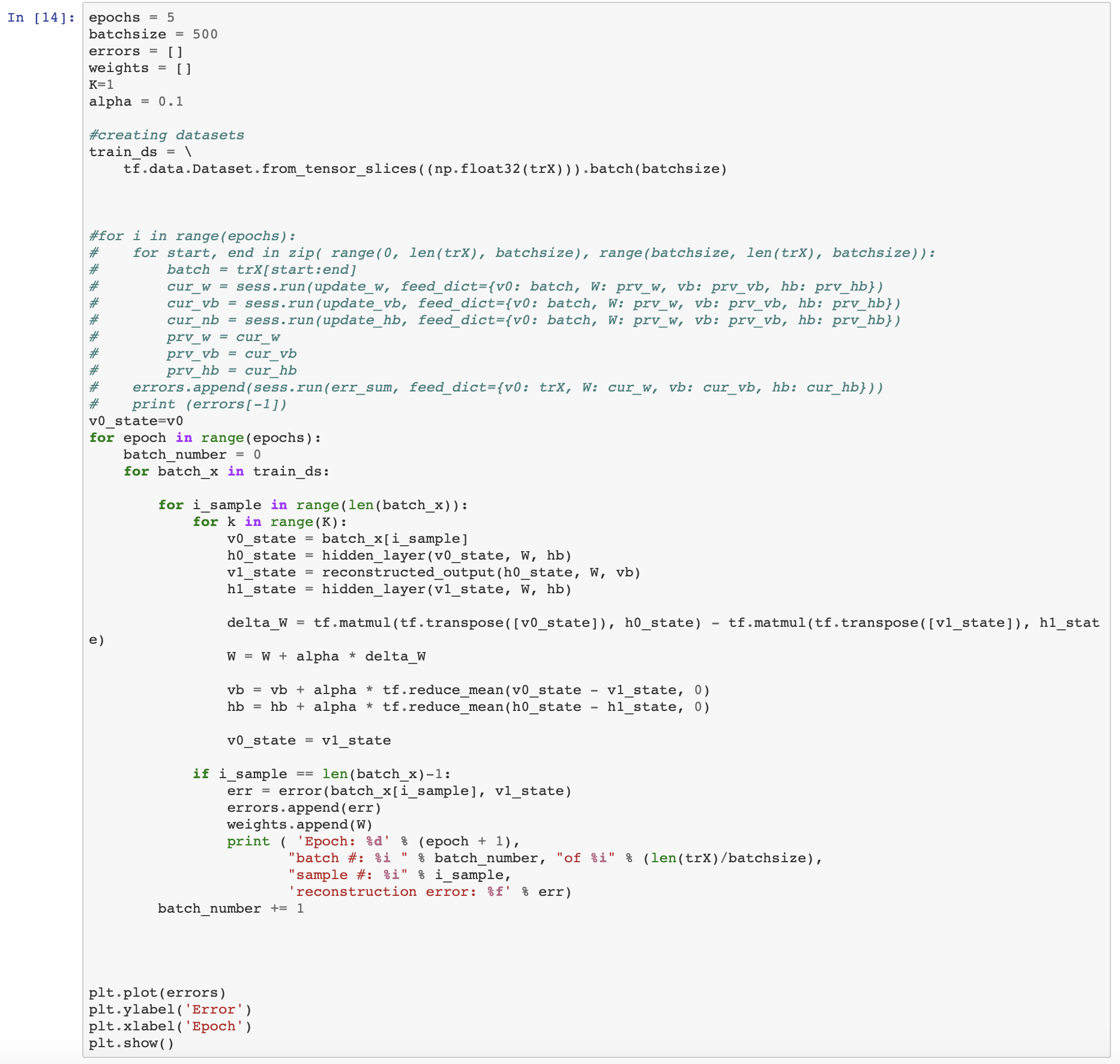You next create a mock user by providing a mock user id and feeding it into the model.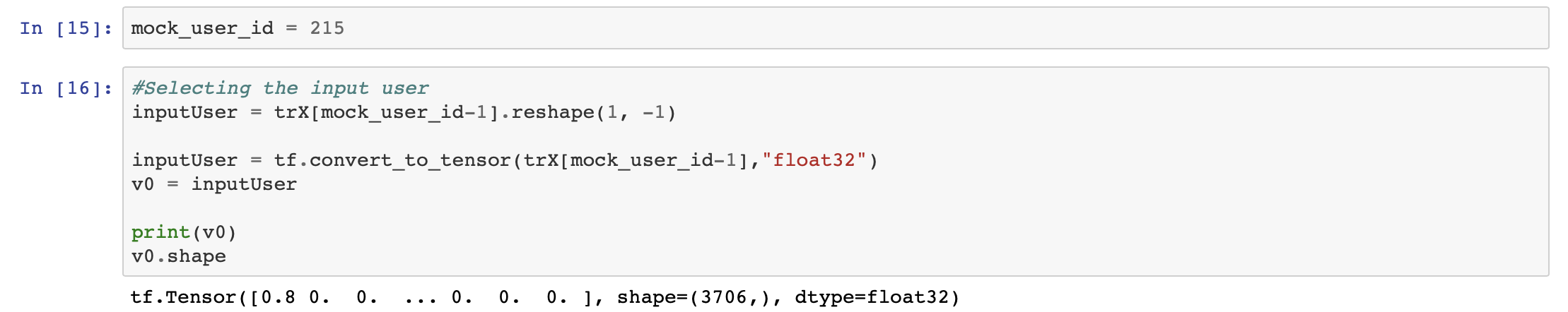Then, list the recommended movies for the mock user. You list the 20 most recommended movies for the mock user by sorting it by their recommendation scores that are provided by the model.You then add a timestamp based on the user using the ratings_df data frame using the following function:

movies_df_mock = ratings_df[ratings_df['UserID'] == mock_user_id]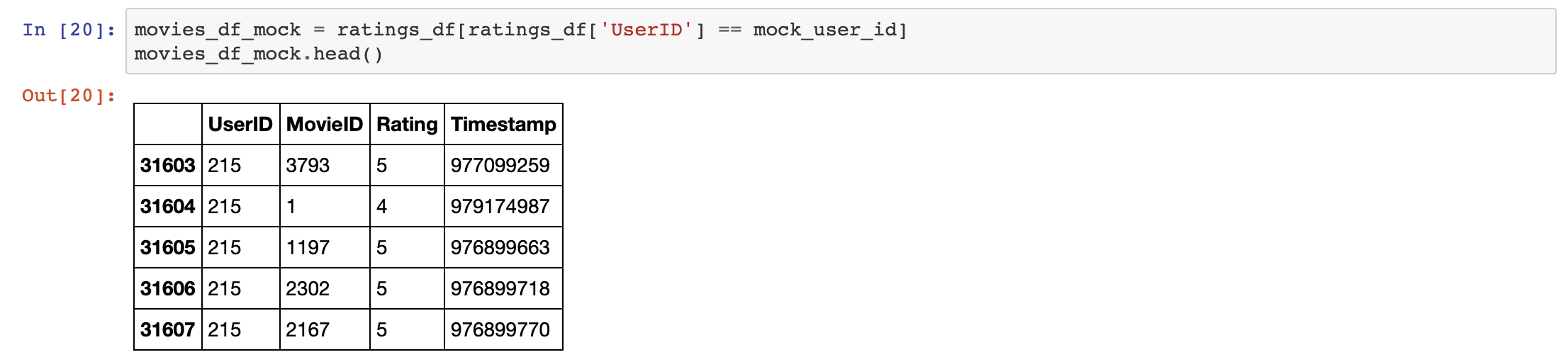Finally, merge the watched movies with the predicted scores. You merge and output the first 20 rows, which let you see the users’ watched movies as well as recommended movies based on their recommendation score.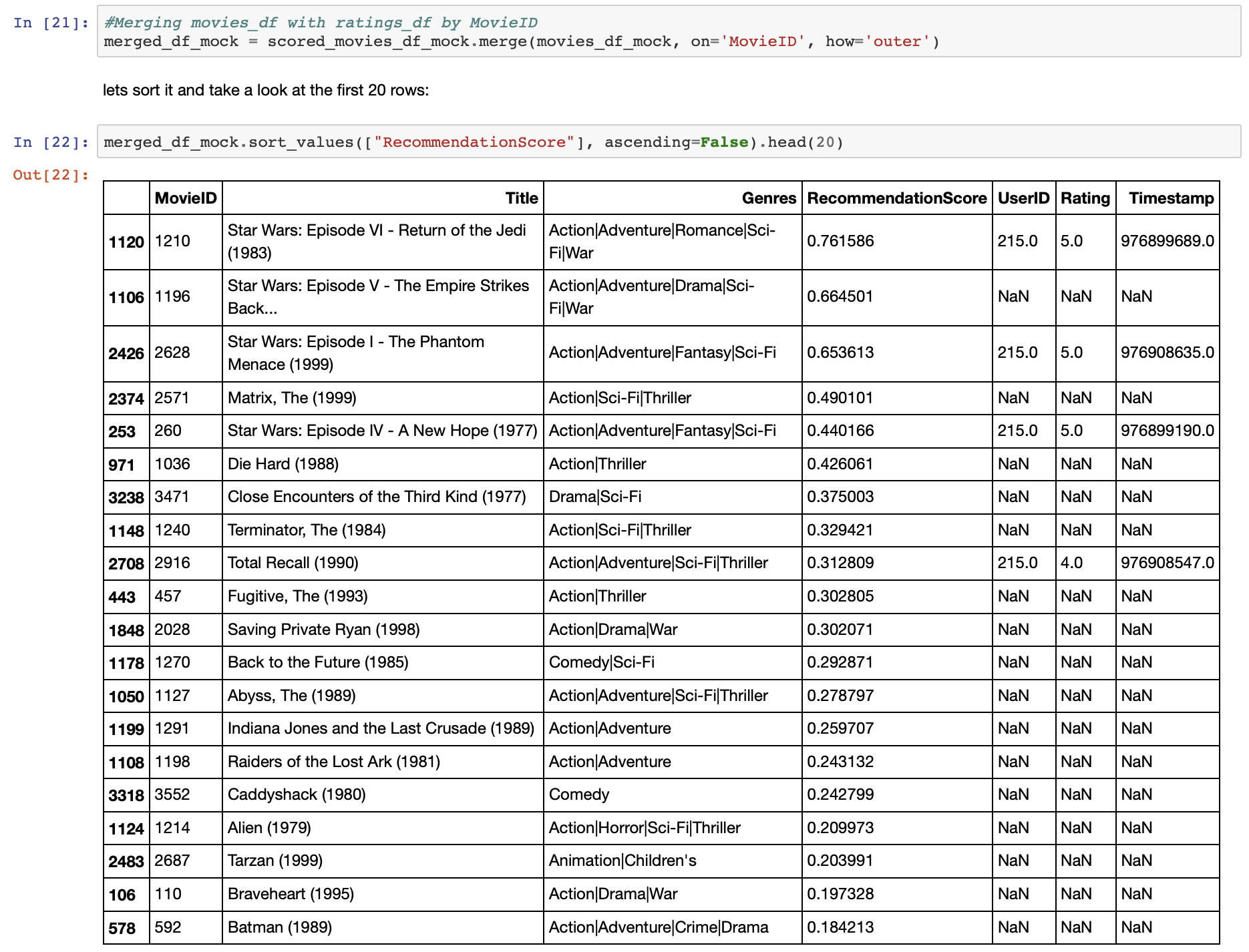I use the watched movies in the recommendation system to indicate what the user has watched already, and based on their watched history make recommendations for movies that they will be more favorable to watch in the future. In other words, letting the user know where the prediction is coming from.

With this last step, you have completed going through the notebook. You can try to change the model parameters like adding more units to the hidden layer or changing the loss functions to see whether anything changes. For more optimization, you can change the number of epochs, the size of K, and the batch size. These are all interesting numbers to explore because they will give a different output.

Summary

In this tutorial, you looked at the basics and implementation of restricted Boltzmann machines using TensorFlow, and created a movie recommendation model based on collaborative filtering, where ratings and users were involved to give the recommendation for the movies a user would be interested to watch.

Legend